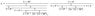# AC Circuit Superposition

• Engineering
• Rurik1
Are you trying to find the current through the 1 Ohm resistor or the voltage across the 1 Ohm resistor?f

## Homework Statement

I need help with determining the current through the 1Ω resistor.

http://img403.imageshack.us/img403/5422/acex22.jpg [Broken]

The trouble I have is that the voltage sources are phased as given in picture, and I got stuck on how to deal with these phases when calculating the current with impendance.

I know I need to count the impendance, but I get the wrong answer when I count the current from every source (superposition theorem).

## Homework Equations

If I look at it through the superposition theorem and current divider rule, I should get the correct answer with regards to current through the 1 Ω resistor.

I am absolutely certain that I don't do the voltage right, i.e. 5 Δ 30 and 5 Δ -30 . Is this correct thinking?

## The Attempt at a Solution

Back to superposition theorem, I can count the total impendance for every voltage source. Then I divide the voltage, example

(5 Δ 30) / Z Δ ω = I

From there I go to the current divider rule and count the current through the resistor per source. This is repeated with the upper source as:

(5 Δ -30) / Z Δ ω = I

------------------

I've handled these problems well without the voltage phases, but I don't think I handle the voltage phases rather well here. I would really appreciate any pointers on how to proceed!

Last edited by a moderator:
Hi Rurik1, welcome to PF.

You can put all the phasor quantities in complex form. The complex math takes care of the phases.

As a suggestion, why don't you convert the voltage sources and their networks up to the 1 Ohm resistor into their Norton equivalents? Those can then be combined into a single Norton source in parallel with that resistor.

gneill

Thanks for your quick reply! Norton is another solution, however I want to see where I am blinding myself here.

OK, superposition, starting from below:

Z1(3Ohms) = 3Δ0o

Z2(5Ohms) = 5Δ0o

Z3(1Ohms) = 1Δ0o

Z4(L) = 2∏*50*0.05Δ90o

From the source below, the current through 1 Ohm should be as per this picture:

And so on... It amazes me that I can't see it... ?!

EDIT: this means, that the next count from the upper source will be 5Δ-30o, so the impendance angle will be subtracted - is this correct?

#### Attachments

•EQ1.jpg
8.6 KB · Views: 415
Last edited:
Your picture looks like an exercise in continued fractionsMy approach would be to work symbolically until the last stage, defining useful intermediate values as required. So, taking your definitions for Z1 through Z4, I'd define Z5 = Z3||Z4 for example. Then to find the current through Z3 due to V2 (the bottom source), after suppressing V1 I'd note the voltage divider consisting of Z2 and Z5. Thus:
$$I_2 = V_2 \left(\frac{Z_5}{Z_2 + Z_5}\right) \frac{1}{Z_3}$$
After that the numbers can be plugged in and the result obtained.

EDIT: this means, that the next count from the upper source will be 5Δ-30o, so the impendance angle will be subtracted - is this correct?

I'm not clear on what you're asking here.